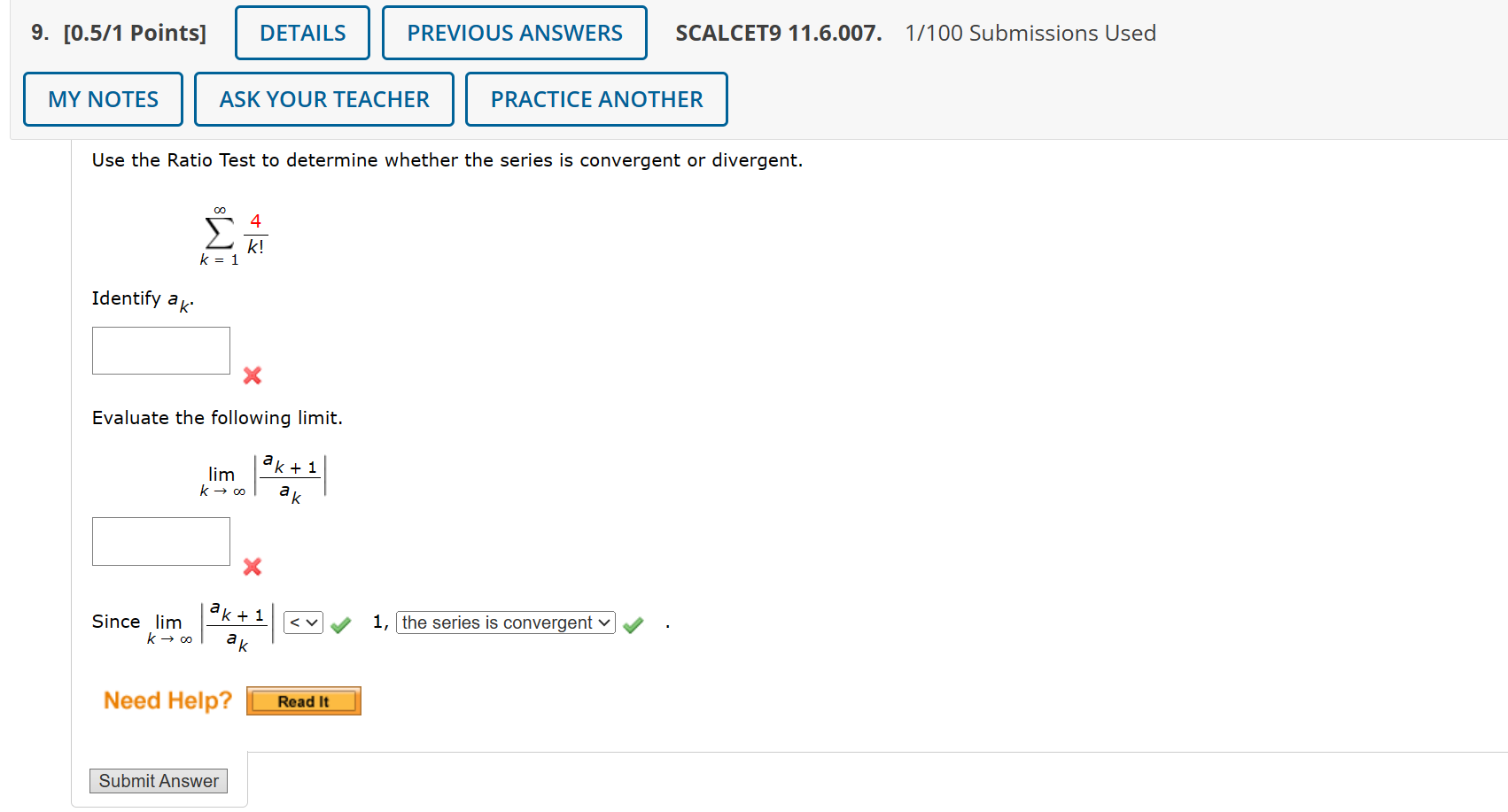Home / Expert Answers / Calculus / 11-6-homework-use-the-ratio-test-to-determine-whether-the-series-is-convergent-or-divergent-k-1-pa536

# (Solved): 11.6 HOMEWORK Use the Ratio Test to determine whether the series is convergent or divergent. k=1 ...

11.6 HOMEWORKUse the Ratio Test to determine whether the series is convergent or divergent. Identify Evaluate the following limit. Since ,

We have an Answer from Expert